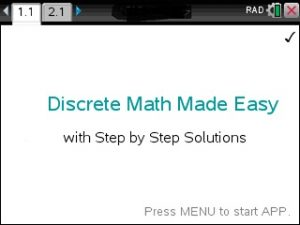## Step by Step Discrete & Finite Math app for the Ti-Nspire CAS CXOverview and Examples at https://youtu.be/8jdUbPRnijs

The most comprehensive Discrete Math APP for calculators.

Step by Step……

• Linear Programming
• Marginal Analysis, Demand Analysis
• Markoff Chains
• Simplex Algorithm
• Probabilities
• Combinatorics
• Functions
• Vector and Matrices
• Solve Equations
• Logic with Set Theory, Truth Tables
• Easy to use. Just plug in the equation and the correct answer shows.
Posted on Categories discrete math